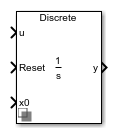# Integrator (Discrete or Continuous)

Discrete-time or continuous-time integrator

• Library:
• Simscape / Electrical / Control / General Control

•## Description

The Integrator (Discrete or Continuous) block implements a simple integrator in conformance with IEEE 421.5-2016.

You can switch between continuous and discrete implementations of the integrator using the Sample time parameter.

### Equations

Continuous

To configure the integrator for continuous time, set the Sample time property to `0`. This representation is equivalent to the continuous transfer function:

`$G\left(s\right)=\frac{1}{s}.$`

From the preceeding transfer function, the integrator defining equations are:

`$\left\{\begin{array}{c}\stackrel{˙}{x}\left(t\right)=u\left(t\right)\\ y\left(t\right)=x\left(t\right)\end{array}\text{\hspace{0.17em}}\text{\hspace{0.17em}}x\left(0\right)={x}_{0},$`

where:

• u is the integrator input.

• x is the integrator state.

• y is the integrator output.

• t is the simulation time.

• x0 is the initial state of the integrator.

Discrete

To configure the integrator for discrete time, set the Sample time property to a positive, nonzero value, or to `-1` to inherit the sample time from an upstream block. The discrete representation is equivalent to the transfer function:

`$G\left(z\right)=\frac{{T}_{s}}{z-1},$`

where Ts is the sample time. From the discrete transfer function, the integrator equations are defined using the forward Euler method:

`$\left\{\begin{array}{c}x\left(n+1\right)=x\left(n\right)+{T}_{s}u\left(n\right)\\ y\left(n\right)=x\left(n\right)\end{array}\text{\hspace{0.17em}}\text{\hspace{0.17em}}x\left(0\right)={x}_{0},$`

where:

• u is the integrator input.

• x is the integrator state.

• y is the integrator output.

• n is the simulation time step.

• x0 is the initial state of the integrator.

### Defining Initial Conditions

You can define the state initial conditions using the input port x0. The integrator state reverts to the initial condition any time it is reset.

### Limiting the Integral

You can limit the integral output using one of two methods:

• Set Limit type to `Anti-windup` to use the anti-windup saturation method.

The anti-windup method limits the integrator state x between the lower saturation limit A and upper saturation limit B:

`$A<=x<=B\text{\hspace{0.17em}}.$`

Because the state is limited, the output can respond immediately to a reversal of the input sign when the integral is saturated.

• Set Limit type to `Windup` to use the windup saturation method.

The windup method limits the integrator output y between the lower saturation limit A and upper saturation limit B:

`$A<=y<=B\text{\hspace{0.17em}}.$`

Because the output is limited, the state can continue to grow when the integrator is saturated. As a result, the output cannot respond to a reversal of the input sign until the state has reached the limiting saturation point.

### Resetting the State

You can reset the state of the integrator by passing a nonzero signal to the Reset port of the block.

## Ports

### Input

expand all

Integrator input.

Data Types: `single` | `double`

Integrator reset. To reset the integrator state to the value of the x0 port, pass a nonzero value to this port. Alternatively, attach a zero-valued Constant block to this port to override the external reset.

Data Types: `single` | `double`

Integrator initial state. To specify the value of the state after a reset, pass a signal to this port.

Data Types: `single` | `double`

### Output

expand all

Integrator output.

Data Types: `single` | `double`

## Parameters

expand all

Select the external reset strategy for the integrator:

• Select `rising` to reset the state when the reset signal rises from a negative or zero value to a positive value.

• Select `falling` to reset the state when the reset signal falls from a positive value to a zero or negative value.

• Select `either` to reset the state when the reset signal changes from zero to a nonzero value, from a nonzero value to zero, or changes sign.

• Select `level` to reset the state when the reset signal is nonzero at the current time step or changes from nonzero at the previous time step to zero at the current time step.

Select the limit type of the integrator:

• Select `Anti-windup` to limit the state of the integrator, preventing windup.

• Select `windup` to limit the output of the integrator, allowing windup of the integrator state.

Integrator upper saturation limit. Set this to `inf` for an unsaturated upper limit, or to a finite value to saturate the integrator using the strategy set by Limit type.

Integrator lower saturation limit. Set this to `-inf` for an unsaturated lower limit, or to a finite value to saturate the integrator using the strategy set by Limit type.

Time between consecutive block executions. During execution, the block produces outputs and, if appropriate, updates its internal state. For more information, see What Is Sample Time? and Specify Sample Time.

For inherited discrete-time operation, specify `-1`. For discrete-time operation, specify a positive integer. For continuous-time operation, specify `0`.

If this block is in a masked subsystem, or other variant subsystem that allows you to switch between continuous operation and discrete operation, promote the sample time parameter. Promoting the sample time parameter ensures correct switching between the continuous and discrete implementations of the block. For more information, see Promote Block Parameters on a Mask.

 IEEE Recommended Practice for Excitation System Models for Power System Stability Studies. IEEE Std 421.5-2016. Piscataway, NJ: IEEE-SA, 2016.